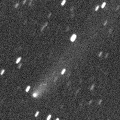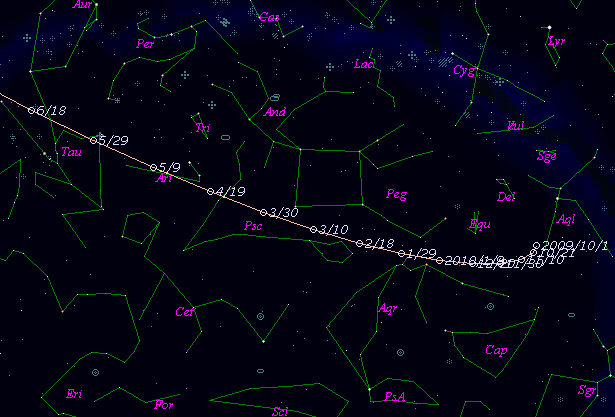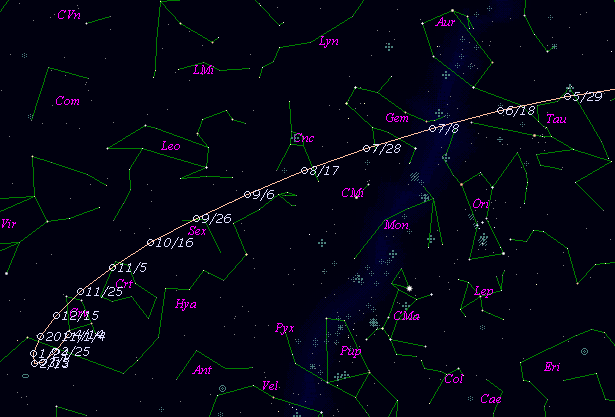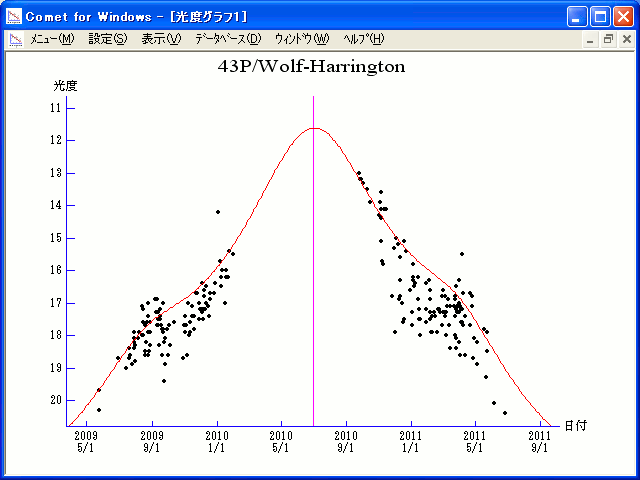# \$B%t%)%k%U(B-\$B%O%j%s%H%sWB@1(B

43P/Wolf-Harrington (2010)###\$B###\$B50F;MWAG(B

```Epoch 2010 June 13.0 TT = JDT 2455360.5
T 2010 July 1.75180 TT                                  MPC
q   1.3576243            (2000.0)            P               Q
n   0.16091436     Peri.  191.46931     +0.15734146     -0.95316168
a   3.3476458      Node   249.89577     +0.92642047     +0.23305968
e   0.5944540      Incl.   15.96650     +0.34203623     -0.19278486
P   6.13
From 2192 observations 1996 Sept. 17-2010 Dec. 7, mean residual 0".7.
Nongravitational parameters A1 = +0.27, A2 = -0.0138.
```

###\$B@1?^(B###\$B8wEYJQ2=(B

```        m1 = 7.5 + 5 log\$B&\$(B + 17.0 log r
```##### \$B50F;MWAG\$O(BM.P.E.C. 2010-X76\$B\$K7G:\\$5\$l\$?\$b\$N\$G\$9!#(B \$B@1?^\$O(B StellaNavigator Ver.2.0 for Windows (\$B%"%9%H%m%"!<%D(B\$BJTCx(B / \$B%"%9%-!<=PHG6I4)(B) \$B\$G:n@.\$7\$?\$b\$N\$G\$9!#(B \$B8wEY%0%i%U\$O(BComet for Windows\$B\$G:n@.\$7\$?\$b\$N\$G\$9!#(B